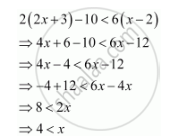CBSE (Arts) Class 11CBSE
Share

# Solve the Given Inequality for Real X: 2(2x + 3) – 10 < 6 (X – 2) - CBSE (Arts) Class 11 - Mathematics

ConceptAlgebraic Solutions of Linear Inequalities in One Variable and Their Graphical Representation

#### Question

Solve the given inequality for real x: 2(2x + 3) – 10 < 6 (x – 2)

#### SolutionThus, all real numbers x,which are greater than or equal to 4, are the solutions of the given inequality.

Hence, the solution set of the given inequality is (4, ∞).

Is there an error in this question or solution?

#### APPEARS IN

NCERT Solution for Mathematics Textbook for Class 11 (2018 to Current)
Chapter 6: Linear Inequalities
Q: 13 | Page no. 122
Solution Solve the Given Inequality for Real X: 2(2x + 3) – 10 < 6 (X – 2) Concept: Algebraic Solutions of Linear Inequalities in One Variable and Their Graphical Representation.
S